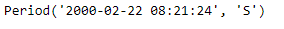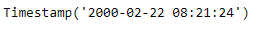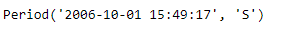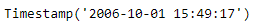Python | Pandas Period.start_time

• Last Updated : 06 Jan, 2019

Python is a great language for doing data analysis, primarily because of the fantastic ecosystem of data-centric python packages. Pandas is one of those packages and makes importing and analyzing data much easier.

Pandas Period.start_time attribute returns a Timestamp object containing the start time of the given period object.

Syntax : Period.start_time

Parameters : None

Return : Timestamp object

Example #1: Use Period.start_time attribute to find the start time for the given Period object.

 # importing pandas as pdimport pandas as pd  # Create the Period objectprd = pd.Period(freq ='S', year = 2000, month = 2, day = 22,                         hour = 8, minute = 21, second = 24)  # Print the Period objectprint(prd)

Output :Now we will use the Period.start_time attribute to find the start time for prd object.

 # return the start timeprd.start_time

Output :As we can see in the output, the Period.start_time attribute has returned a Timestamp object which contains the date value as well as the start time of the given period object.

Example #2: Use Period.start_time attribute to find the start time for the given Period object.

 # importing pandas as pdimport pandas as pd  # Create the Period objectprd = pd.Period(freq ='S', year = 2006, month = 10,                hour = 15, minute = 49, second = 17)  # Print the objectprint(prd)

Output :Now we will use the Period.start_time attribute to find the start time for prd object.

 # return the start timeprd.start_time

Output :As we can see in the output, the Period.start_time attribute has returned a Timestamp object which contains the date value as well as the start time of the given period object.

Attention geek! Strengthen your foundations with the Python Programming Foundation Course and learn the basics.

To begin with, your interview preparations Enhance your Data Structures concepts with the Python DS Course. And to begin with your Machine Learning Journey, join the Machine Learning - Basic Level Course

My Personal Notes arrow_drop_up